Quantities and Symbols Definitions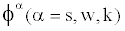Volume fractions of solid network, water and ionic species N Total number of ionic species E Elastic strain vector of solid phase t Time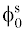Solid-phase volume fraction at a reference configuration Ck Concentration of the kth ionic species Mk Molar weight of the kth ionic species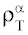True mass density of phase α vα Velocity vector of phase α.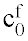Fixed charge density at reference configuration.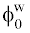Water volume fraction at reference configuration σ Mixture stress μα(α=s,w,k) Chemical potential of phase α fαβ = fβα Frictional coefficients between phases α and β zk(k=1,2,3,…N) Valence of ion α p Fluid pressure I Identity tensor Tc Chemical expansion stress Φk Osmotic coefficient of kth ionic species λs, μs Lame coefficients of solid matrix γk Activity coefficient of the kth ionic species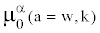Chemical potential of phase α at reference configuration R Universal gas constant T Absolute temperature Fc Faraday constant ψ Electrical potential Bw Coupling coefficient.
Table 1: The parameter definitions of the multi-phasic mixture theory.
Goto home»